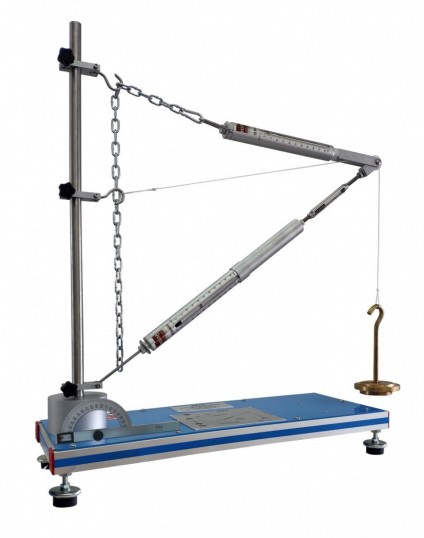# MFPG Unit for studying Forces in a Jib Crane혁신적인 시스템

The Unit for studying Forces in a Jib Crane, "MFPG", is designed to study the tensile and compressive forces in a planar central force system based on the example of a crane jib.

일반적인 설명보기

실험실

관련 뉴스

#### 일반적인 설명

The Unit for studying Forces in a Jib Crane, "MFPG", is designed to study the tensile and compressive forces in a planar central force system based on the example of a crane jib.

The unit represents a planar central force system in which multiple forces act on a single point of application. It consists of an adjustable length bar and a chain, which make the jib of the crane. This jib is attached to a vertical member (support bar) by adjustable clamp elements.

A weight holder and a set of weights apply loads to the jib of the crane.

The force of the bars is measured with two spring balances installed on the adjustable length bar and the chain (elements that represent the jib).

Based on the example of a crane jib, forces are determined experimentally, theoretically and graphically: cable force, tensile force and compressive force. The magnitudes and directions of the forces are graphically determined with a force parallelogram.

### 매뉴얼에 포함 된 가이드 실기 연습

1. Study of the force parallelogram.
2. Graphical breakdown of forces by force parallelogram.
3. Determination of the bar forces on various jib forms: cable force, tensile force and compressive force.
4. Comparison of results obtained in the practical exercises, the theoretical values and the values obtained from the graphical method with the force parallelogram.
5. Comprehension of the action of the crane cable forces on the jib and the effect of the jib inclination.

#### 품질#### 판매 후 서비스